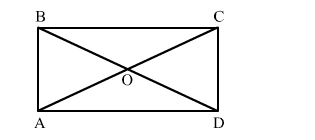# A diagonal of a rectangle is inclined to one side of the rectangle at 35°.

Question:

A diagonal of a rectangle is inclined to one side of the rectangle at 35°. The acute angle between the diagonals is
(a) 55°
(b) 70°
(c) 45°
(d) 50°

Solution:Given: In rectangle ABCD, ∠OAD = 35°.

"> ∠OAB = 90° -">- 35° = 55°

In ΔOAB,

Since, OA OB      (Diagonals of a rectangle are equal and bisect each other)

"> OAB = OBA = 55°     (Angles opposite to equal sides are equal)

Now, in ΔODA,

55° + 55° + ∠DOA = 180°       (Angle sum property of a triangle)

"> DOA = 180° -">- 110°

"> DOA = 70°

Thus, the acute angle between the diagonals is 70°.

Hence, the correct option is (b).